MAGNETISM an essential topic in COORDINATION CHEMISTRY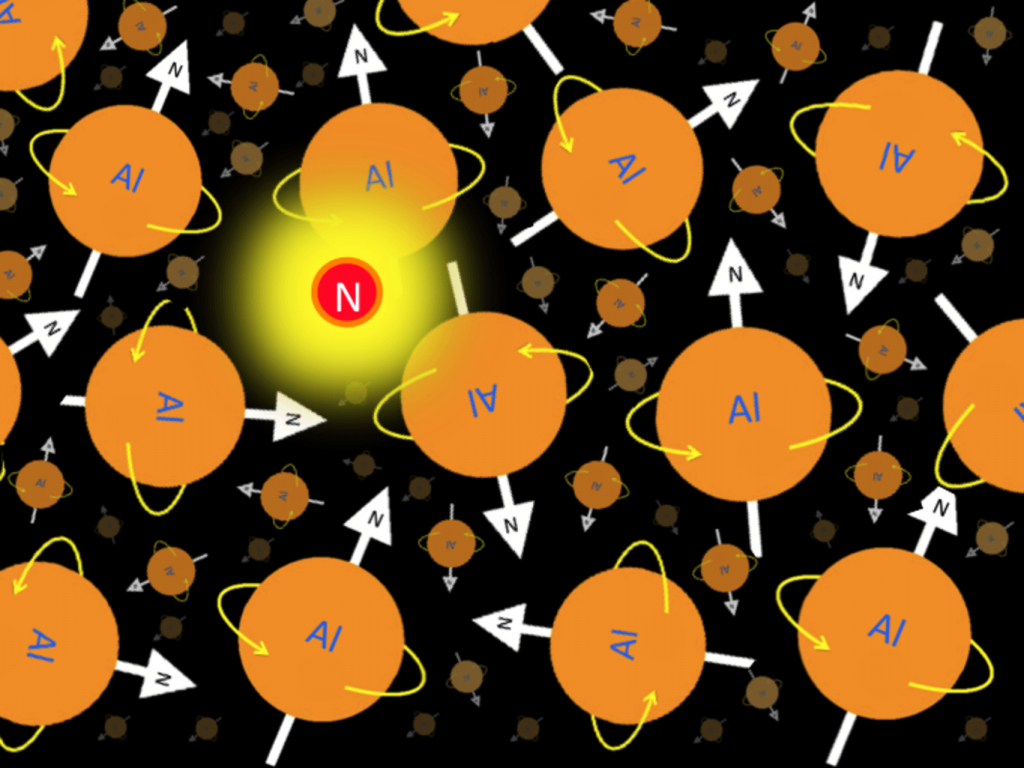Electronic spectroscopy is a powerful method for investigating transition metal complexes. Additional and complementary information can be provided by magnetic measurement because complexes generally have partially filled metal d or f orbitals so a large range of magnetic properties can be expected depending on oxidation state, e– configuration & co-ordination number of central atom.

DIAMAGNETISM

• When a substance is placed in an external or applied magnetic field, there is an induced circulation of e– in substance, this induced circulation of e– give rise to a magnetic moment or magnetic field and if this opposes the applied magnetic field then the substance is repelled by the magnetic field.
• This effect is called Diamagnetic effect.
• This effect is caused by the presence of paired electron.
• The diamagnetic effect exists only when a substance is placed in the magnetic field.
• The substances that have only paired electron exhibits a weak magnetic moment called Diamagnetism and such substances are called Diamagnetic Substance.

If a substance has only paired e–, the diamagnetic effect is dominated while if a substance has paired as well as unpaired electron, the paramagnetic effect is dominated”.

Diamagnetism is a universal property of matter since all compound contains some paired electron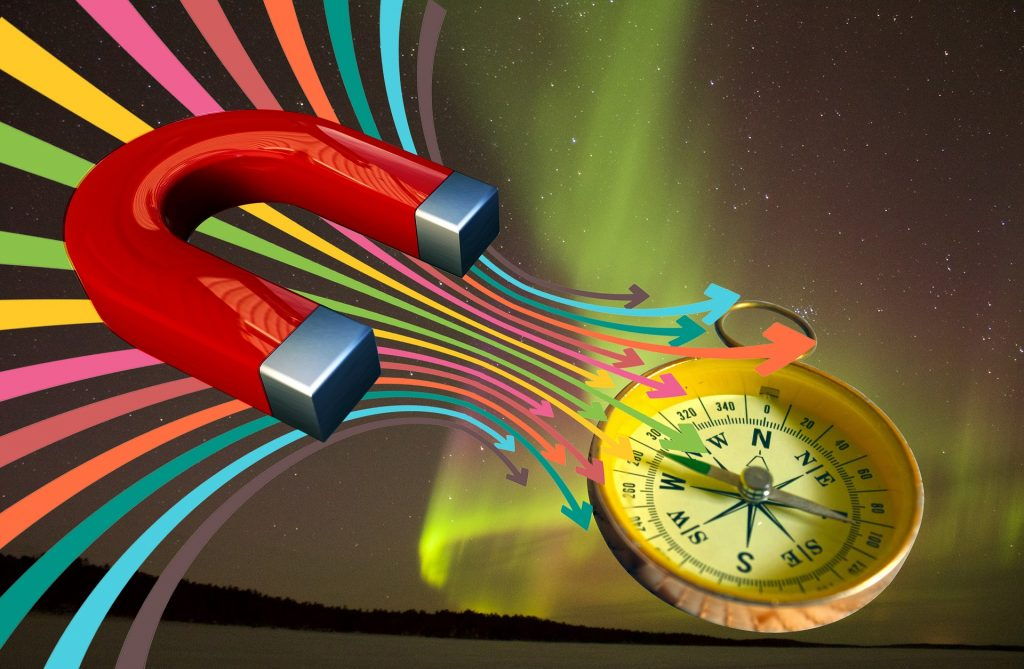PARAMAGNETISM:-

• Any substance that has one or more unpaired e¬– exhibits stronger & permanent magnetic property known as paramagnetism
• Paramagnetism arises from spin and orbital motion of unpaired e– in the absence of external magnetic feild

When a paramagnetic substance is placed in an external magnetic field, the permanent magnetic moments tends to align themselves in the direction of external magnetic field and as a result they are attracted into the magnetic fields.

Since, paramagnetic effect in much large than diamagnetic effect & opposite to diamagnetic effect, it cancels the diamagnetic effect.

• Thus even substances having only one unpaired electron per molecule will show a net attraction into a magnetic feild.

The paramagentic effect is observed only in the persence of an external field. When field is removed, individual molecular moments are randomised by thermal motion and bulk sample has no overall moment. When a field is present there is a competition between the thermal tendency towards randomness & field capacity to force alignment. As a result, paramagnetic effects decrease in magnitude as temperature is increased. It is due to the fact that increase in temperature, increase randamization and decreases paramagnetic effect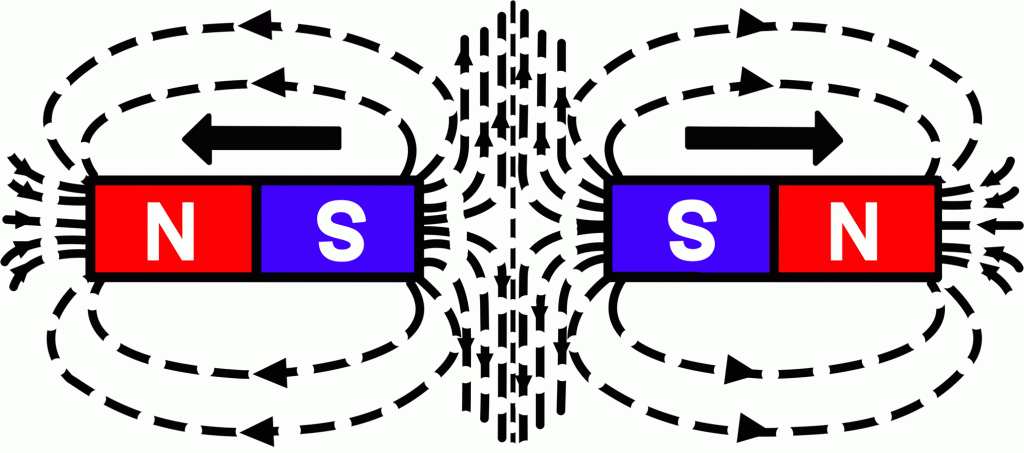Now, When any substance is placed in a magnetic field, the field produced within the sample will either be greater than or less than applied field, depending on whether the material is paramagentic or diamagnetic.
The difference between the two is expressed as:-Where B is induced field inside the sample,  is external field or free field value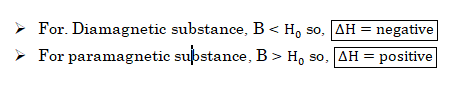More commonly, the difference between the applied and induced field in sample is expressed in terms if I (ie. intensity of magnetisation), which is magnetic moment per volume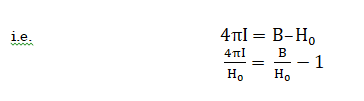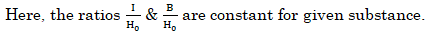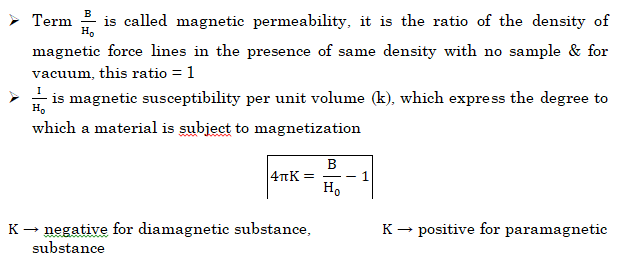The quantity that is most frequently obtained from experimental measurement for magnetism is specific or mass susceptibility.

It is related to volume susceptibility through density (d)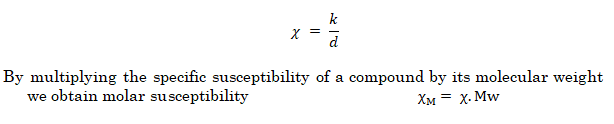• Methods in laboratory for the measurement of magnetic susceptibility

(a) Gouy Method

MORDERN TECHNIQUE:-    SQUID (Super conductor quantum interference device)

• Once an experimental value of has been obtained for a paramagnetic substance it can be used to determine how many unpaired e are there per-molecule or ion.
• Next step is to connect the macroscopic susceptibility to individual molecular moment & finally to unpaired electron.
• From classical theory, the corrected or paramagnetic molar susceptibility is related to permanent magnetic moment of molecule (μ).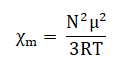R is ideal gas constant
T is absolute temperature
μ is expressed in Bohr Magneton (1BM= eh/4πm)

Now, solving this expression for magnetic moment we get:-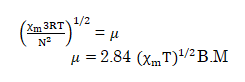• Pierre curie has shown that the paramagnetic susceptibility is inversely proportional to absolute temperature.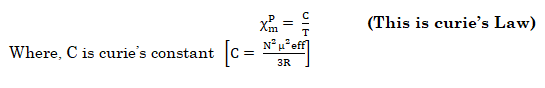This law is valid only for paramagnetic substances that are magnetically dilute (those in which paramagnetic centers are well separated from each other) by diamagnetic atoms.

• NOTE:- Magnetic susceptibility χ of a material is a measure of how easy it is to align electron spins with the applied field.
•           A paramagnetic material has positive susceptibility.
•           A Diamagnetic material has negative susceptibility.

Cooperative Mechanism

• The materials that are not magnetically dilute, unpaired spins on neighboring atoms may couple with each other, this phenomenon is called Magnetic Exchange.
• These substance may behave as either ferromagnetic or ferromagnetic or antiferromagnetic and they follow Currie-Weirs Law (modification of curie’s Law) (This is called cooperative magnetism).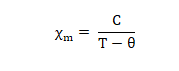Where, Θ is a constant with units of temperature.

• NOTE– When the paramagnetic species are very close together (as in bulk metal or are separated by species that can transmit interactions (as in many d-block metal oxides flourides & chlorides), the metal centers may interacts (couple) with one another, this give rise to ferro or anti-ferro.
• Note: The Paramagnetism is hot topic for “CSIR NET Coaching in Chemistry”.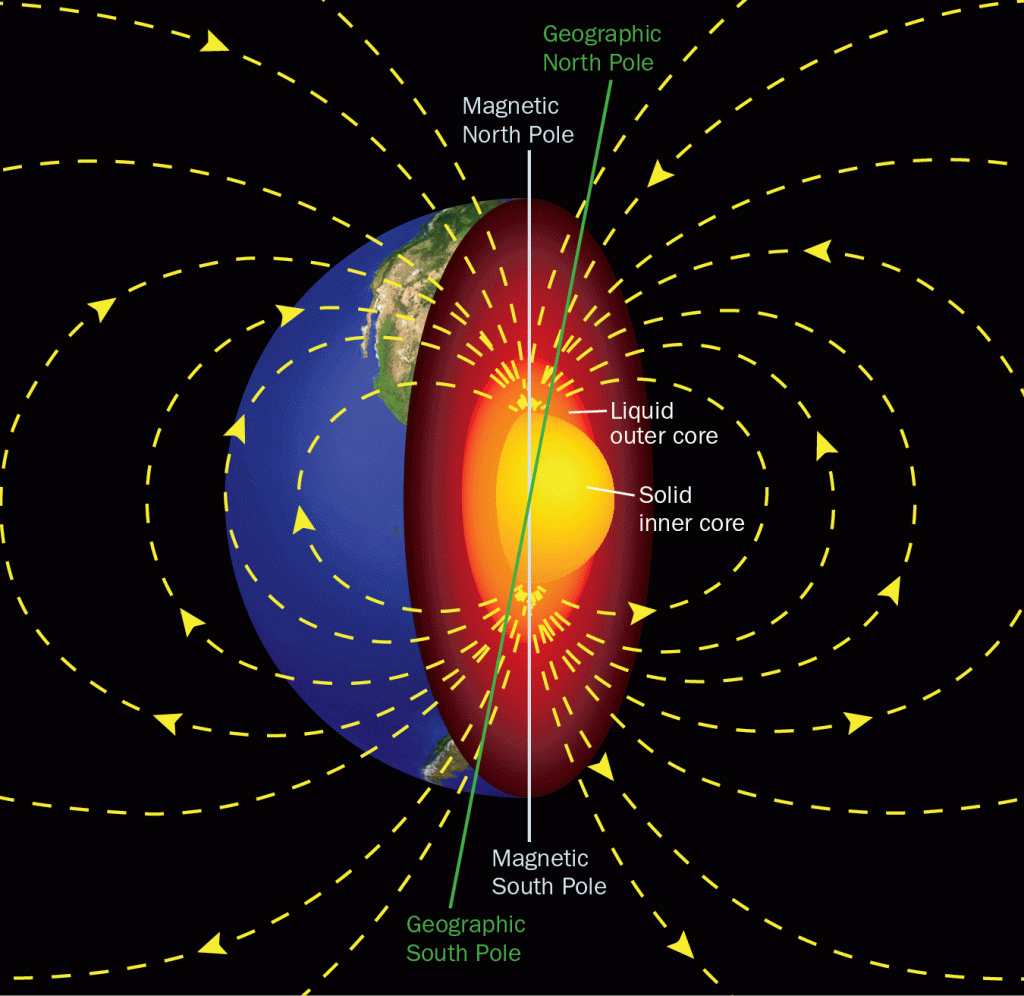Ferromagnetism:-

• If the interacting magnetic dipoles on neighboring atom tends to assume a parallel alignment, substance is said to be ferromagnetic.
• Ferromagnetism leads to greatly enhanced para magnetism as in iron metal at temperature of up to 1043 K above which thermal energy is sufficient to overcome the alignment and normal paramagnetic behavior prevails
• On cooling a sample, ferromagnetic ordering (change from paramagnetic to ferromagnetic occurs at curie temperature and curie’s weirs law is written as:-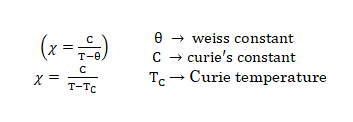• If there is interaction between the magnetic moment on neighboring atoms of a paramagnetic substance, spontaneous ordering of magnetic moment occur below a particular temperature, called as critical temperature.
• If alignment of all the magnetic moment of neighboring atoms is in same direction & produce a permanent magnetic moment substance is said to be ferromagnetic and critical temperature is called curie temperature ( Tc) .

Above this temperature, the substance behaves as a normal paramagnetic substance below Tc, the ferromagnetic substance obeys Curie Weiss law,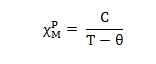For example Fe, Co, Ni & CrO2 exhibit ferromagnetism below curies temperature (1043K, 1404K, 631K & 386K)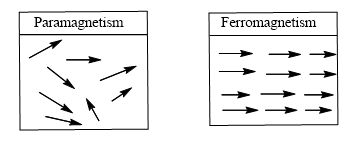Note: Paramagnetism and Ferromagnetism are very useful for “GATE COACHING in Chemistry”.

Antiferromagnetism

If there is a tendency for anti–parallel arrangement of coupled spins, then the substance is said to be Antiferromagnetic.

If half of the magnetic moments are alighned in opposite direction to the other half resulting in a net zero magnetic moment, the substance is said to be anti–ferromagnetic and the critical temperature is called Neel Temperature.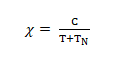Where, TN is Neel temperature

An example of Antiferromagnetism is MnO which has NaCl type structure & has Neel temperature of 118K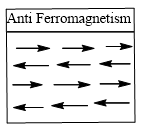Ferrimagnetism

If some of the magnetic moments are systematically aligned opposite to the others to give resultant magnetic moment and the critical temperature is called Curie temperature T_C.

Ex. Magnetite (Fe3 O4), In this substance, the magnetic moments of Fe(II) & Fe(III) are aligned in opposite directions and resultant magnetic moment is only from Fe(II) moment.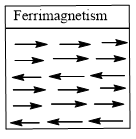Plot of reciprocal of paramagnetic susceptibility v/s absolute temperature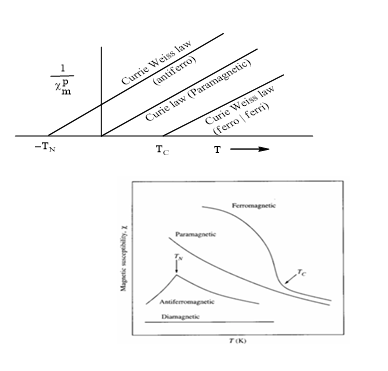Variation of magnetic susceptibility with temperature for diamagnetic, paramagnetic, ferromagnetic, and antiferromagnetic substances. Transition to paramagnetic behavior for ferromagnetic and antiferromagentic substances occur at the Curie (Tc) and Neel (TN) temperatures, respectively.

Note: Paramagnetism, Ferromagnetism, Antiferromagnetism and Ferrimagnetism are essential topics for “JAM COACHING in Chemistry”.

Super exchange:- When a bridging ligand facilitates the coupling of electron spins on adjacent metal centers, the mechanism is called super exchange.
In super exchange pathway, the unpaired e– on the first metal centre M1 interacts with a spin–paired pair of e– on bridging ligand with the result that unpaired e– on M2 is aligned in an antiparallel manner with respect to M1.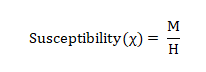Where, M is magnetization produced,   H is Field applied

Ex. MnO, NiO occurs to give rise to Anti ferromagnetism)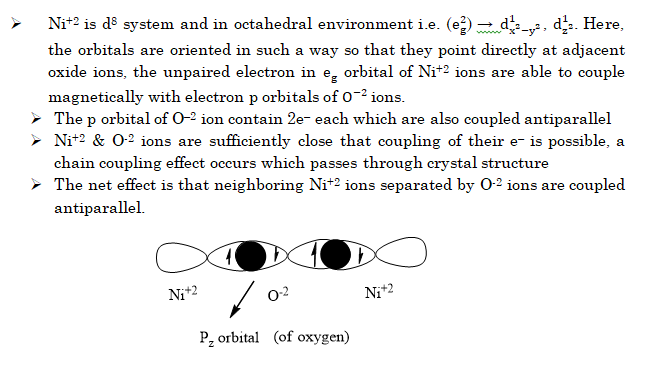The above mentioned topic is essential for CSIR-NET Coaching in Chemistry. It is a hot topic for “GATE Coaching in Chemistry” and JAM COACHING in Chemistry

Introduction of Coordination chemistry, an essential Branch of Inorganic Chemistry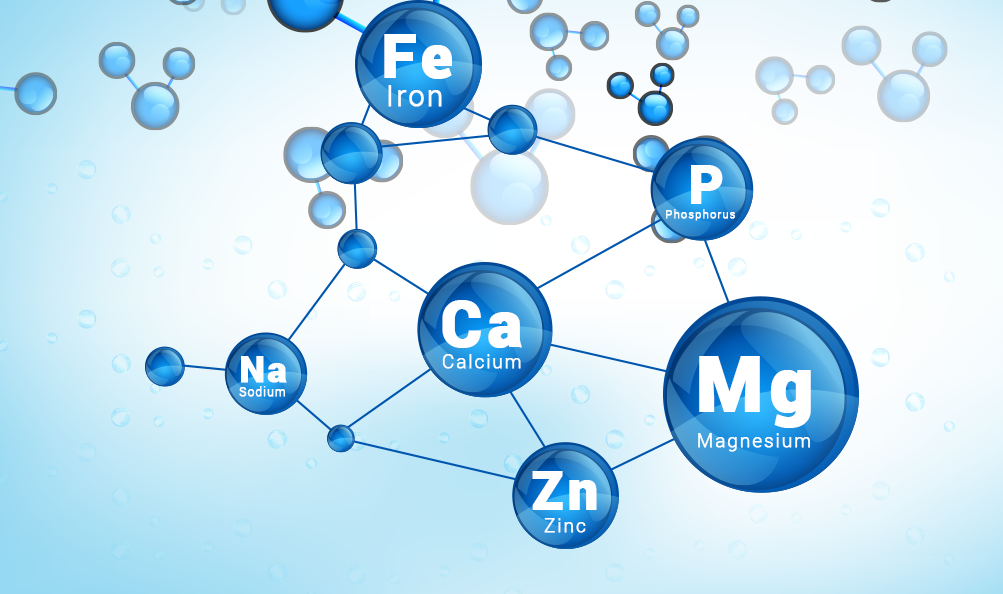The branch of Inorganic Chemistry that deals with the study of coordination compounds is called coordination chemistry.

A compound in which a metal cation or atom is attached to a group of ligands by coordinate bond is called co-ordination complex.

Ligand: Any species (ion or molecule) that have atleast one lone pair of electrons and have tendency to donate it to metal cation or atom. It is electron rich species that is why it is called as Lewis base (or nucleophile).

Metal: It is an electron deficient species which can accept a pair of electrons. Therefore, it behaves as lewis acid or an electrophile. A group of ligands donate its pairs of electrons. One donor atom of a ligand donates to metal cation to form coordinate bond. The product so formed is known as coordination compounds. These compounds may be neutral or ionic compounds as: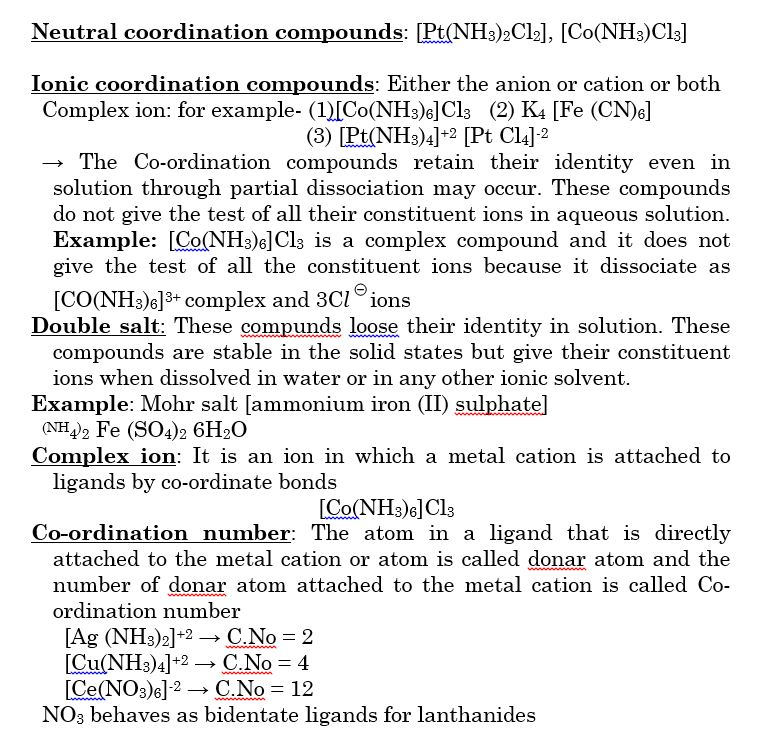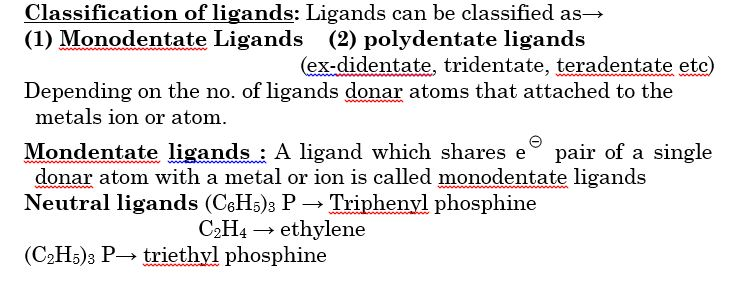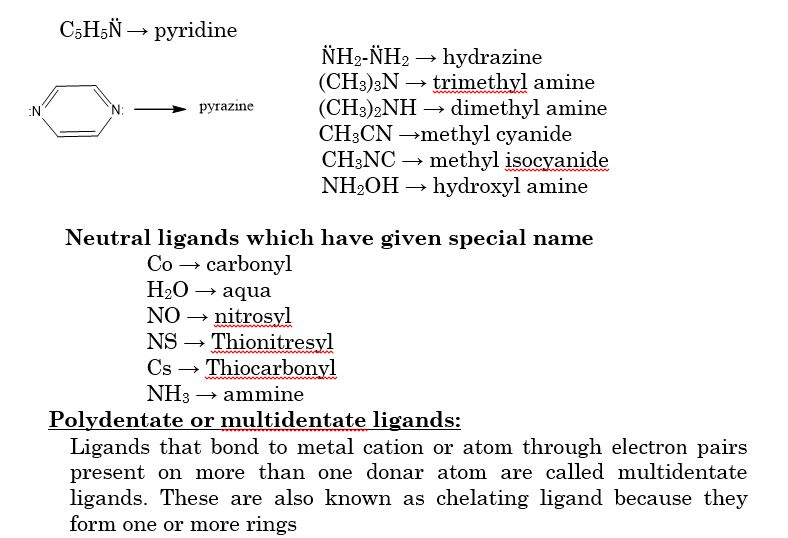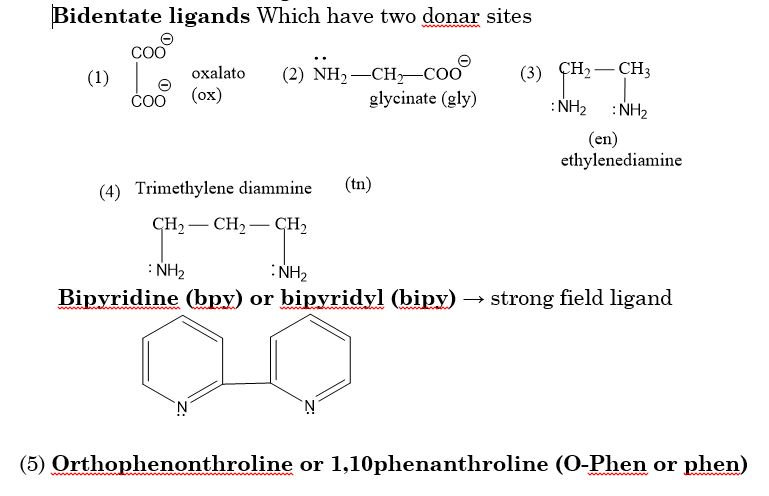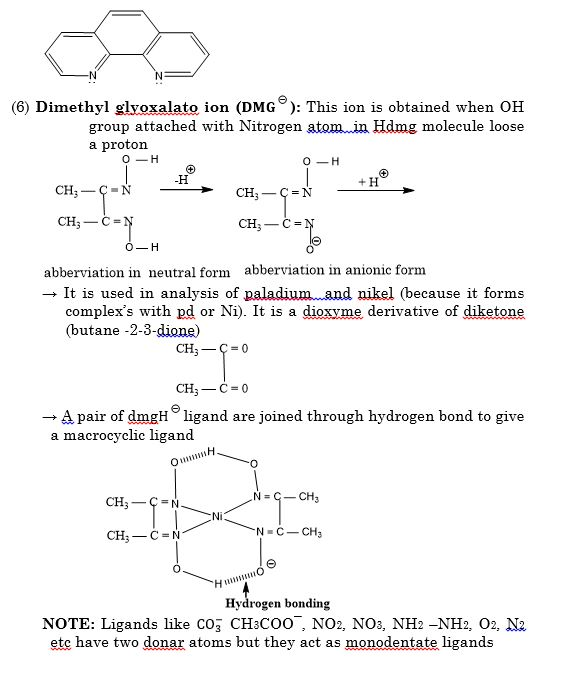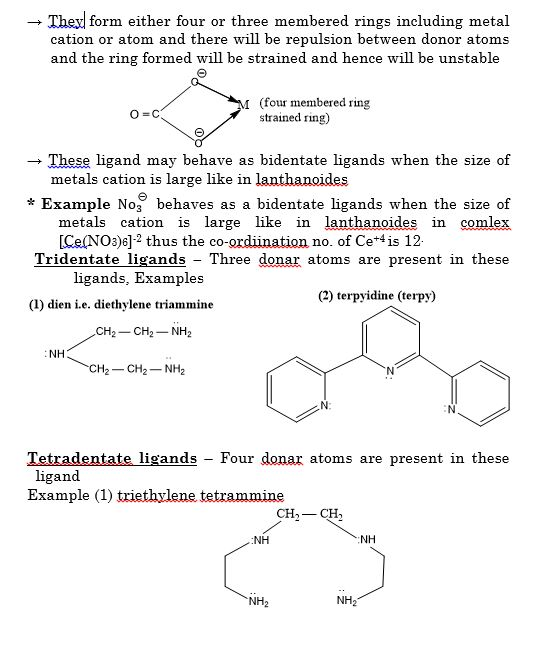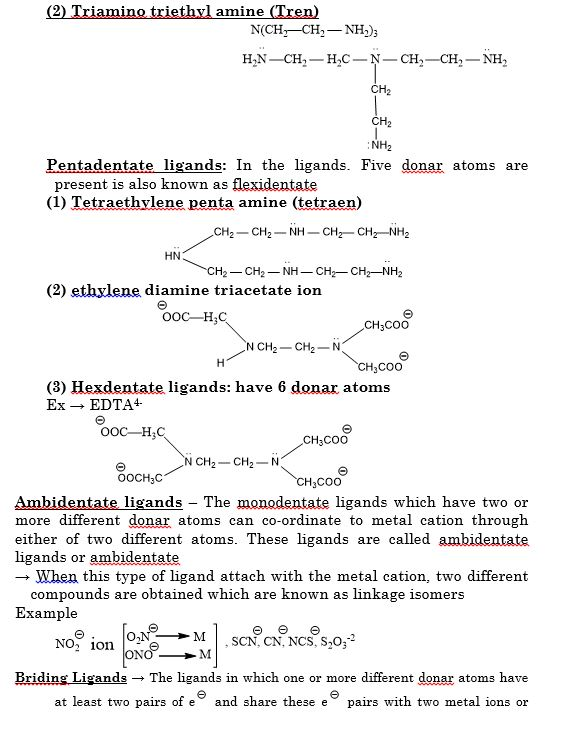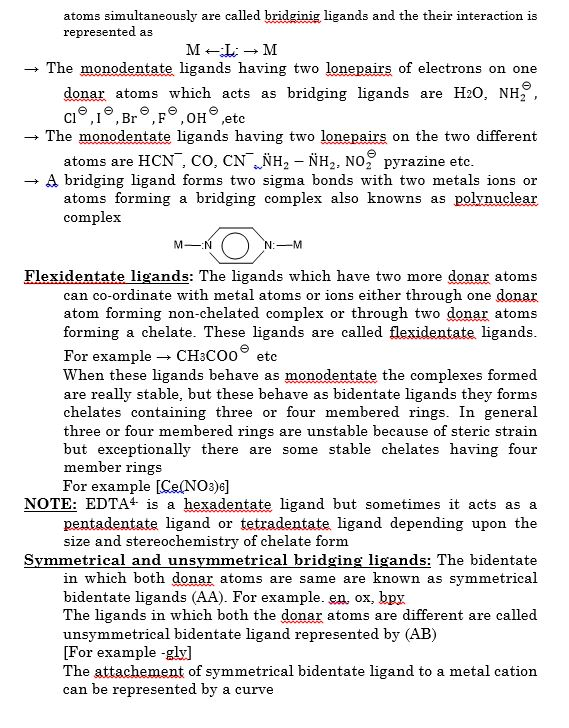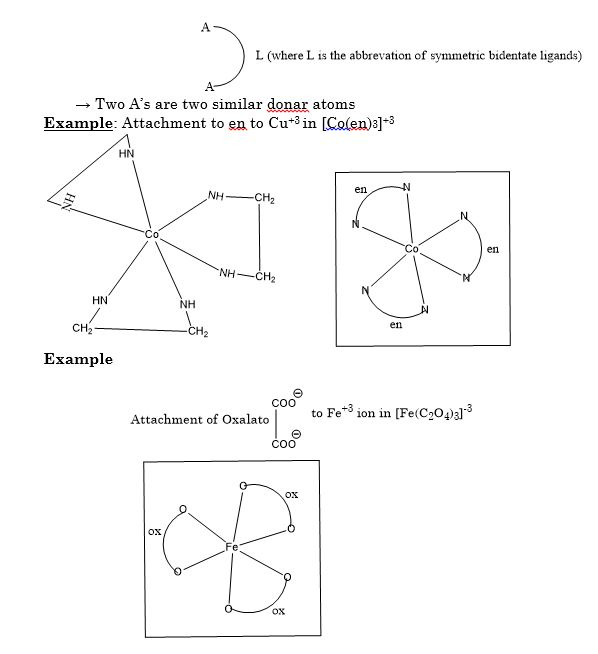Dependence of Rate of Reaction in CHEMICAL KINETICS, an innovative topic of Physical Chemistry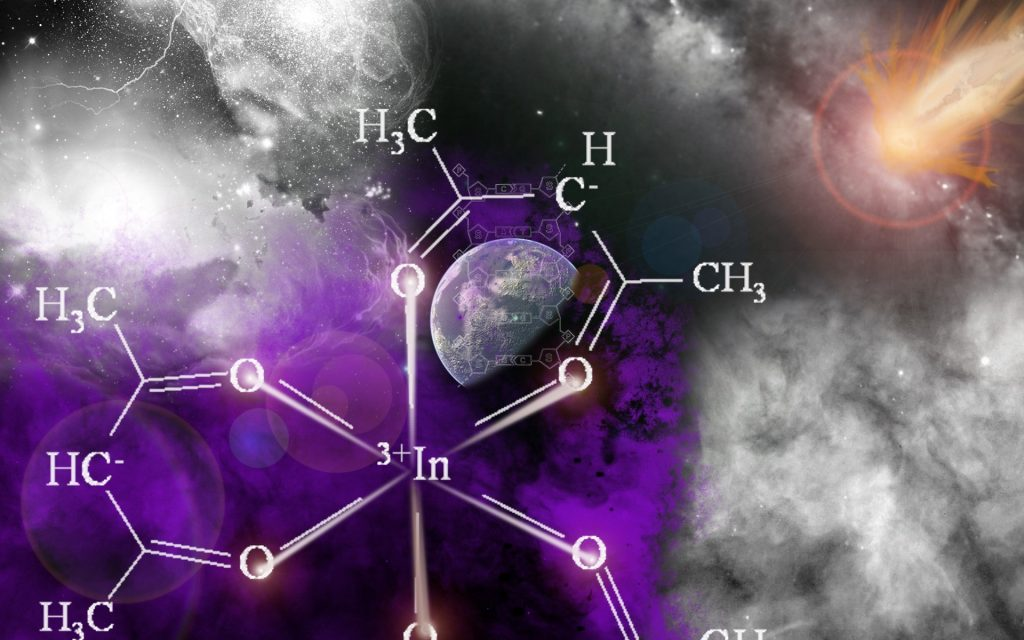Dependence of Reaction Rates on concentration (Rate law expression)

When a chemical reaction proceeds, concentration of reactant decreases while that of products increases.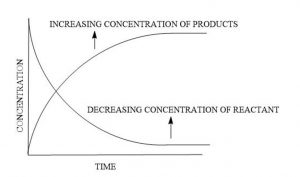→ It is indicated from the fig, In the biginning, only reactants are present so that the concentration of product is Zero
→As the reaction proceeds, the concentration of the reactants decreases and that of preoduct increases.
→At the start of the reactant, rate of reaction is large and it decrease with passage of time. It means that rate of reactant is directly proportional to concentration of reactants. To increase the rate of a reactant we should increase the concentration of one or all reactants and vice.versa.
→In the gas phase, the increse in pressure of the reactant gases increase in pressure, the number of molecule per unit valume increase and therefore, rate of reactant increase.

Law of Mass Action

→The quantitave relationship between the rate of a reaction and the molar concentration of the reacting substance was given by Norwegian Chemists, Guldbege and waage. This relationship is called Law of mass action
→According to his law; At a given temperature the rate of chemical reactant is directly proportional to the product of the molar concentration of the reactants. The molar concentration of reactants is also called active mass.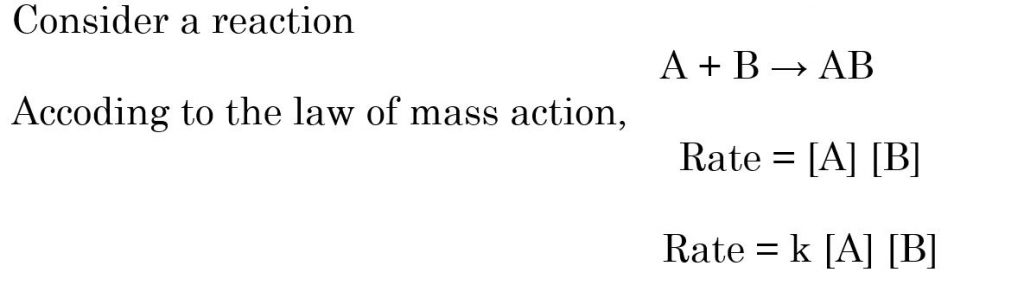where [A] and [B] are molar concentration of [A] and [B]
K → Proportionality constant, called rate contant, also called velocity constant
If [A] = [B] = 1 (unity) then rate = K
Thus rate constant of a reactnat at given temperture may be defined a rate of reaction when concentration of each of the reactant is taken as unity

Characteristic of a Rate constant

The rate constant has the following properties-
(i) Rate constant is a measure of rate of reaction
Larger the value of K , Faster is the raection
Smaller the value of K , Slower is the reaction
(ii) Different reactnt have different value of K
(iii) At a fixed temperture, the value of K constant and is characteristic of the reaction. However, it changes with temperature
For the particular order reactant, the rate constant is independent of concentration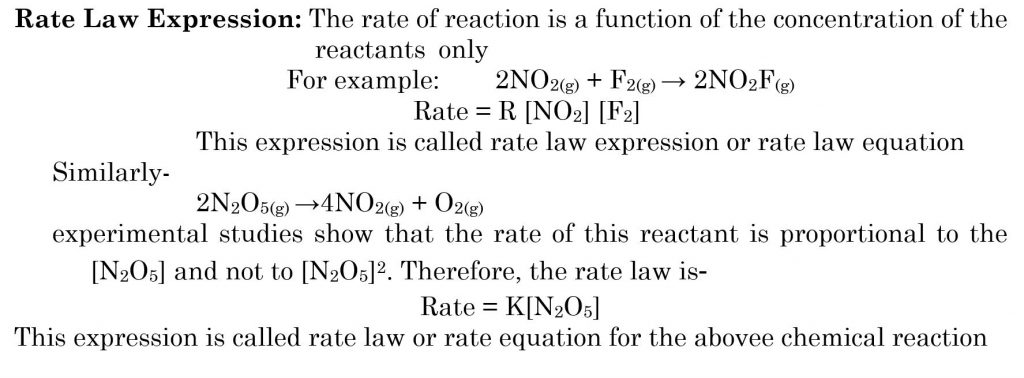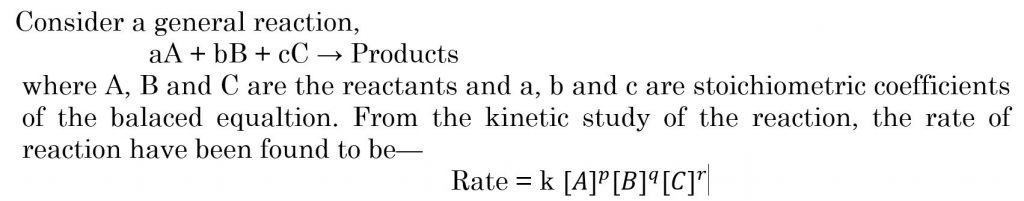where p, q, and r are constant numbers or the power of the concentration of the reactant A, B and C respectively, on which the rate of reactant depends
—The values p, q and r are determined experimentally and may or may not be equal to a, b and c
—The mathemical expression which denotes the experimentally observed rate of a reaction in terms of the concerntrations of the reacting species which influences the rate of reactant is called rate law or rate equation.
— The power of concentration of reactnts in rate law expession may or may not be same as the coeefucient of reactant in the balanced chemical equation.
— On the other hand., the law of mass action given the rate expression on the basis of overall balanced equation.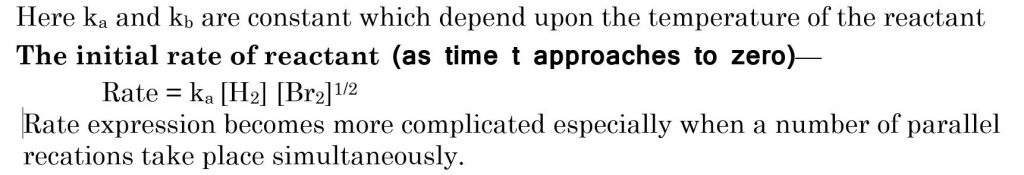All the topics studied under Chemical Kinetics are very useful for CSIR NET coaching in Chemistry, GATE coaching in Chemistry and IIT JAM coaching in Chemistry.

CHEMICAL KINETICS – An Essential Branch of Chemistry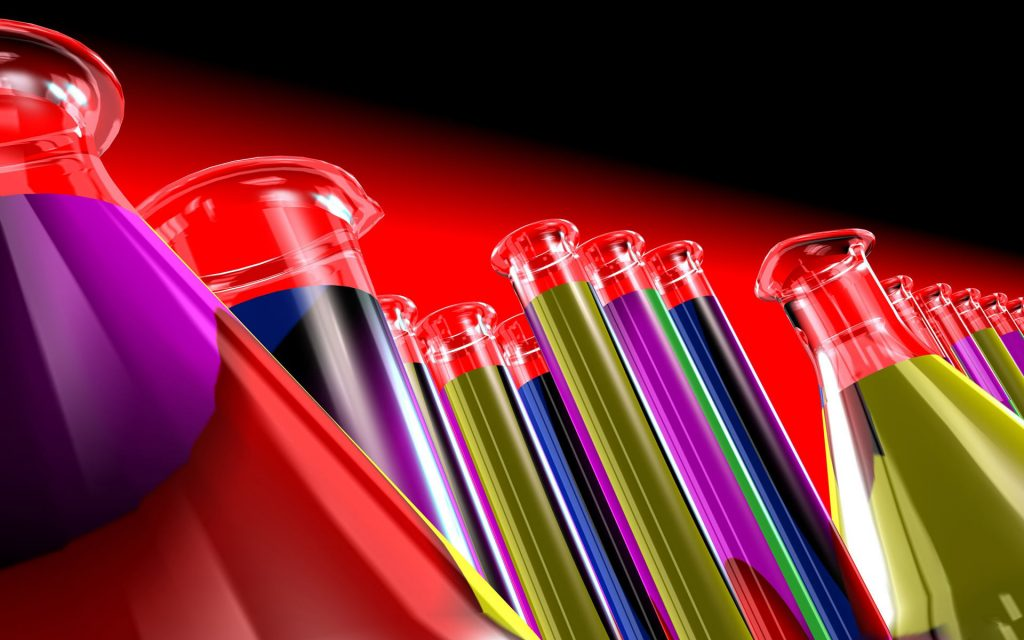The feasibility of a given reaction can be predicted with the help of thermodynamic principles. Besides this, the relative amounts of reactants and products at equilibrium position of the reaction can also be predicted. In addition, we can predict whether changes in the experimental conditions will increase or decrease the amount of a product at equilibrium. However, thermodynamic principles do not provide any information regarding the speed of the given reaction, i.e. how much time a given feasible reaction will take for its completion. Moreover, it is not possible to predict how the speed of a given reaction gets affected by changing the experimental conditions. The study of rate of reactions including its dependence on the concentrations of reacting species and the experimental conditions constitutes one of the topics of physical chemistry and is known as chemical kinetics. The important application of the study of rate of reaction is to use the kinetic data in establishing the molecular pathway or mechanism by which the reaction takes places. This branch of chemistry is very useful for CSIR NET coaching in Chemistry, GATE coaching in Chemistry and IIT JAM coaching in Chemistry.

Rate of Reaction

The rate of a chemical reaction is a measure of the speed with which the reactants are converted into products. It may be defined as the change in any one of the reactants or products per unit timeIn this reaction, in unit volume, one mole of the reactant (‘R’) produces one mole of the product ‘P’. The rate of reaction may be expressed by the following two ways ______
(i) The rate of disappeorance or decrease in concentration of R (reactant)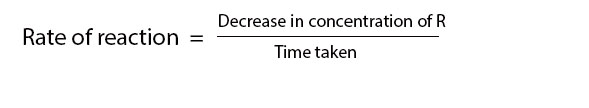(ii) The rate of increase in concentration of P (products)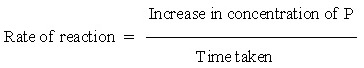If [R1] and [P1] are concentration of R & P at time t1 and [R2] and [P2] are concentration at time t2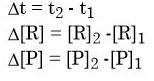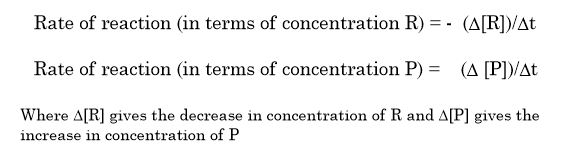Where ∆[R] gives the decrease in concentration of R and ∆[P] gives the increase in concentration of P
The square brackets arround the substances are used to express the molar concentration (mol/litre or mole litre-1)
→ In case of concentration of reactants, we use minus sign
This implies that the concentration of reactants of decreasing with time
∆[R] → negative value
rate always → positive so
we inlude minus sign in the expression of rate with respect to reactant
The above rate is called average rate of the reaction. It has the dimensions of concentration/time. So the unit of rate of reaction will be concentration time-1
if unit of concentration is → mole litre-1 (Molarity)
and unit of time → second
then unit of rate of reaction will be → Mole litre-1 s-1
→ However in gaseous reactions, when concentration of gases is expressed in terms of their partial pressure, then unit of rate of reaction → atmosphere s-1
→ In the above example the stoichimetric coefficients for the reactants and products are same

A + B → 2C

In this case, one mole of A reacts with one mole of B to form 2 moles of C. This means that the rate of disappearance of A and B are same but the rate of disappearance of C is the twice the rate of disappearance of A and B. Thus,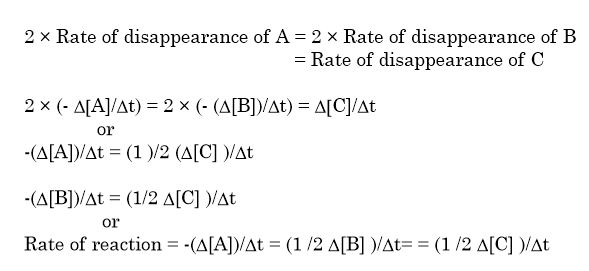e.g. For the decomposition of gaseous nitrogen pentaoxide (N2O5) as –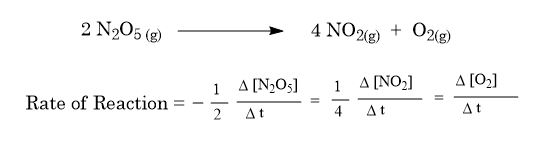Average Rate and Instantantaneous Rate
→ The rate expressions given the average rate of reactions over the time interval (∆t). This is expressed as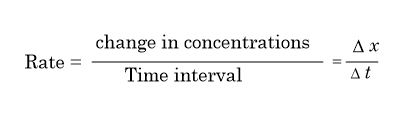→ This concept is similar to mechanical speed.
→ In case of reactions the rate depends upon the concentration of the reactants. As the reaction proceeds, the concentration of the reactants decreases with time. Thus, the rate of reaction may not be constant in the time interval which we measure. Thus, the rate cannot be determined simply by dividing the total change in concentration by the time taken as in case of mechanical speed.
→ On the other hand, rate of reaction may be expressed at a particular moment of time thus, rate of reaction may be defined as the rate of change of concentration of any one of the reactants or products at a given time. Such a reaction rate is also known as instantaneous rate.
→ Mathematically, instantaneous rate may be represented by-(d[R])/dt or (d[P])/dt instead of – (∆[R])/∆t or (∆[P])/∆t respectively. Here d[R] or d[P] represents infinitesionally small changes in concentration of R or P in infinitesimally small interval of time, dt. Thus average rate approches the instantanious rate as ∆t becomes smaller and smaller i.e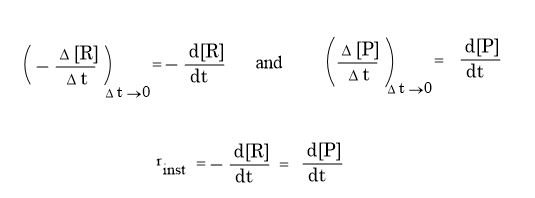In general, if dx represents very small (infinitesimally small) change in concentration o any species during the very small (infinitesimally small) change of time, at the rate of reaction can be expressed as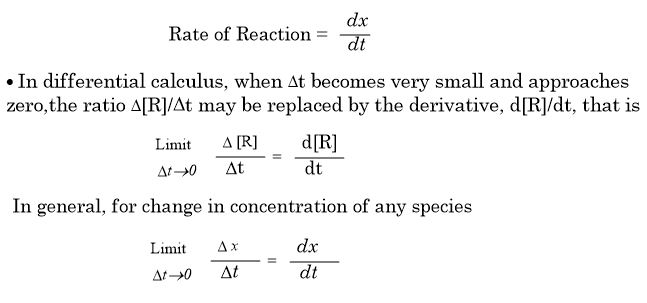where dx is the infinitesimally small change in concentration corresponding to small change in time, dt.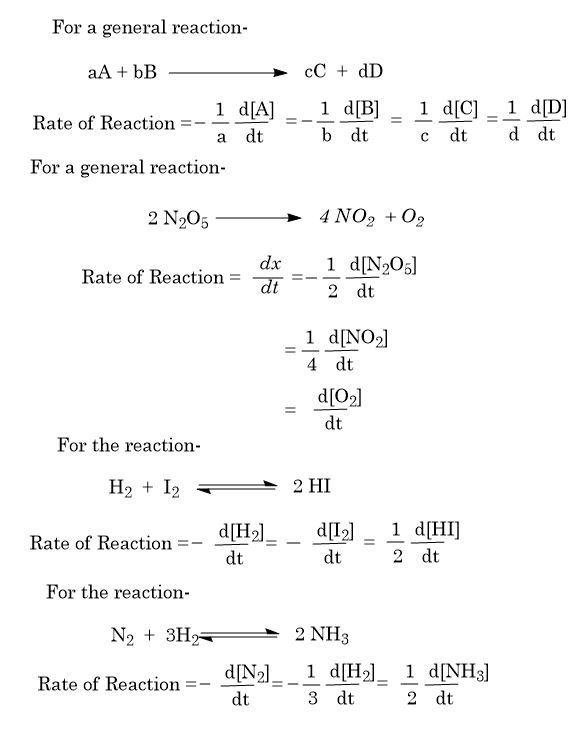All the topics studied under Chemical Kinetics are very useful for CSIR NET coaching in Chemistry, GATE coaching in Chemistry and IIT JAM coaching in Chemistry